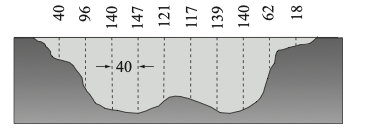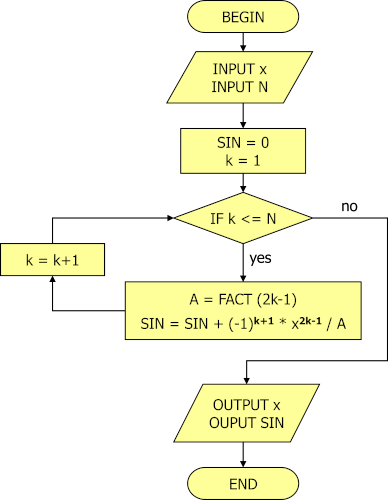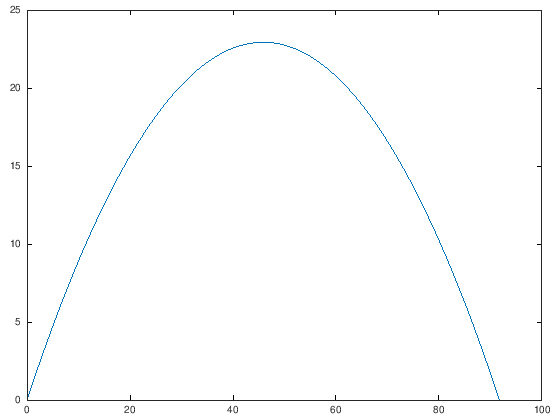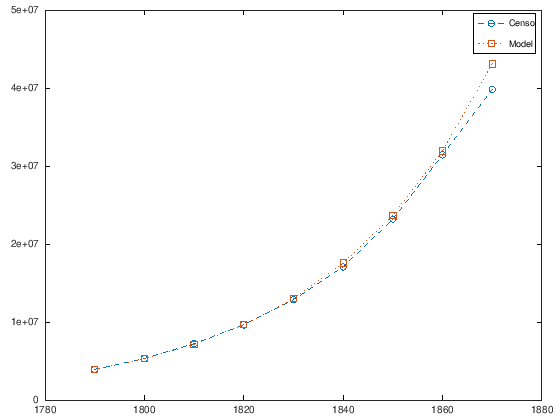CoCalc Public Filesintro_to_numerical_analysis.slides.html
Author: Anonymous User-1597356567
Views : 203
Compute Environment: Ubuntu 18.04 (Stable)
intro_to_numerical_analysis slides

# ISW2101 Métodos Numéricos¶

Prof. Mario González, Ph.D. en InformáticaWeb page

# Welcome to numerical analysis in Octave and Python¶

• What is numerical analysis?
• Have you perform any type of numerical analysis?
• Have you used an old school calculator?
• What was the accuracy?
• Examples of numerical anaylsis.

# Numerical Analysis¶

Numerical Analysis: refers to the analysis of mathematical problems by numerical means, especially mathematical problems arising from models based on calculus.

## Definition and requirements¶

Numerical Analysis: refers to the analysis of mathematical problems by numerical means, especially mathematical problems arising from models based on calculus.

Effective numerical analysis requires several things:

• An understanding of the computational tool being used, be it a calculator or a computer.
• An understanding of the problem to be solved.
• Construction of an algorithm which will solve the given mathematical problem to a given desired accuracy and within the limits of the resources (time, memory, etc) that are available.

## Theoretical, experimental and computational approaches¶

• Traditionally, engineering and science had a two-sided approach to understanding a subject: the theoretical and the experimental. More recently, a third approach has become equally important: the computational.
• Traditionally we would build an understanding by building theoretical mathematical models, and we would solve these for special cases.
• But more practical situations could seldom be handled by direct means, because the needed equations could be too difficult to solve.
• Thus we also used the experimental approach to obtain better information about the system we want to study.
• The theory would suggest ideas to be tried in the laboratory, and the experimental results would often suggest directions for a further development of theory.

### Computational Science¶

• With the rapid advance in powerful computers, we now can augment the study of a problem by directly solving the theoretical models of its domain applied to more practical situations; and this area is often referred to as computational science.
• At the heart of computational science is numerical analysis; and to effectively carry out a computational science approach to studying a physical problem, we must understand the numerical analysis being used, especially if improvements are to be made to the computational techniques being used.

### Computational X¶

• Computational Mathematics
• Computational Physics
• Computational Biology
• Computational Neuroscience
• Computational Finances
• Computational Managemet Science
• Computational Social Science

# Why study numerical methods?¶

• Critical Loads for Buckling a Column.

• Static Analysis of a Scaffolding

• Analysis of Natural Frequencies of a Vibrating Bar.

• Stability of frameworks under external forces (bridges, houses, ...). Mostly numerical linear algebra, sometimes differential equations are solved.

• Communication/power: (i) Network simulation and (ii) Train and traffic networks.

See:

## Example¶

• A cross section of a river with measurements of its depth at intervals of 40 ft is shown in the figure. Use numerical integration to estimate the cross-sectional area of the river.## Why are we taking this course? For SE, TI students.¶

We will improve our:

• Problem solving abilities.
• Learn languages for numerical computation, will be helpful for AI/Data Science applications.
• Do some computer science.Plotting a random series in:

# What are we going to learn?¶

• Within this course, we attempt to show the spirit of the subject.
• Most of our time will be taken up with looking at algorithms for solving basic problems such as:
• matrix algebra and linear systems,
• rootfinding,
• interpolation,
• and differential equations;
• but we will also look at the structure of computers and the implications of using them in numerical calculations.
• We will aim linking at the relationship of numerical analysis to the larger world of science and engineering.

# 􏰐 Expectations¶

• Students enrolling in this course should have competent programming skills to implement computer code of several tens of lines in length, be aware that the workload in this course is non-trivial. 􏰐
• Plagiarism and cheating will be considered an academic offense. 􏰐
• Students are expected and required to have completed the assigned readings in advance of each class.

# Programming¶

• Algorithm: Sequence of logical steps required to perform a specific task.
• Pseudocode: English (natural language) description of a program.
• Flowchart: Visual / graphical representation of a program.## Example: Calculate the $sin(x)$¶

• Calculators either use the Taylor Series for sin/cos or the CORDIC algorithm. A lot of information is available on Taylor Series, so I'll explain CORDIC instead.Check this answer.
$sin(x) = x - \frac{x^3}{3!} + \frac{x^5}{5!} - \frac{x^7}{7!} + \dots$

Algorithm

Step 1: Enter $x$ and the number of terms to use, $N$.

Step 2: Calculate $sin(x)$ using the Taylor series.

Step 3: Print the result.

## Pseudocode¶

INPUT x, N
SIN = 0
LOOP k from 1 to N
SIN = SIN + (-1)^(k+1) * x^(2k-1) / (2k - 1)!
END
OUTPUT x, SIN


## Flowchart¶## Programming Errors (Bugs)¶

• Syntax error: an error in the source code of a program, e.g. writing primtf instead of printf.
• Run-time error: the program stop running at some point, e.g. division by zero or trying to read from a non-existing file.
• Logical error: the program will run, but the result is wrong, e.g. in the sin(x) example taking all the terms as positive.

• Clarity
• put header (author, date, version, purpose, variable definitions, etc.)
• put comments to explain variables, purpose of code segments, etc.
• Testing
• run with typical data
• run with unusual but valid data
• run with invalid data to check error handling

# Which languages and IDEs will we use?¶

• Basic programming skills that you studied in ISW-1102
• MATLAB/Octave and Python 3 syntax$^*$
• Data types (integers, single vs. double precision, arrays, etc.)
• loops, if statements
• input / output
• etc.

$^*$ Octave and Python are interpreted languages.

# A simple example of a numerical model¶

• A mathematical model is a mathematical description of a physical situation.
• By means of studying the model, we hope to understand more about the physical situation. Such a model might be very simple. For example, $A=4 \pi R_e^2,\ R_e \approx 6.371km$ is a formula for the surface area of the earth. How accurate is it?
1. First, it assumes the earth is sphere, which is only an approximation. At the equator, the radius is approximately 6,378 km; and at the poles, the radius is approximately 6,357 km.

2. Next, there is experimental error in determining the radius; and in addition, the earth is not perfectly smooth.

• Therefore, there are limits on the accuracy of this model for the surface area of the earth.
• What was the error in calculated area?

### Projectile motion¶

$x = v_{0x} t = v_0 t cos(\theta)$$y = v_{0y} t - \frac{1}{2} g t^2 = v_{0} t sin(\theta) - \frac{1}{2} g t^2$
• Time of flight or total time of the whole journey
$T = \frac{2 v_0 sin(\theta)}{g}$
In :
function [x y] = projectile(deg=45, v=30)
theta = deg / 180.0 * pi;  % angulo de salida radianes
T = 2*v*sin(theta) / g;  %  tiempo de vuelo
t = linspace(0, T, 100);
x = v * t * cos(theta);
y = v * t * sin(theta) - 0.5 * g * t.^2;
endfunction

[x, y] = projectile();
plot(x, y)

Out:In :
disp("Malthusian Growth Model\n")

Year = 1790:10:1870;

Actual = [3929214 5308483 7239881 9638453 12866020 17069453 23191876 31433321 39818449];

Model(1) = 3929214;
for i=2:9
Model(i) = Model(i-1)*1.349;
endfor

disp([Year' Actual' Model' abs((Model - Actual)./Actual)'*100])

Malthusian Growth Model

1790.00000    3929214.00000    3929214.00000          0.00000
1800.00000    5308483.00000    5300509.68600          0.15020
1810.00000    7239881.00000    7150387.56641          1.23612
1820.00000    9638453.00000    9645872.82709          0.07698
1830.00000   12866020.00000   13012282.44375          1.13681
1840.00000   17069453.00000   17553569.01662          2.83615
1850.00000   23191876.00000   23679764.60341          2.10370
1860.00000   31433321.00000   31944002.45001          1.62465
1870.00000   39818449.00000   43092459.30506          8.22235

In :
plot(Year, Actual, '--o');
hold on;
plot(Year, Model, ':s');
legend('Censo','Model')

Out:Solution of Discrete Growth Model

There are not many discrete models that have an explicit solution. However, it is easy to solve the discrete Malthusian growth model. From the model above, we see that:

$P_1 = (1 + r)P_0,\ P_2 = (1 + r)P_1 = (1 + r)^2P_0, \dots, P_n = (1 + r)P_{n-1} = (1 + r)^nP_0.$$P_n = (1 + r)^nP_0$

This shows why Malthusian growth is also known as exponential growth. The solution to the model that is given by the equation above is an exponential function with a base of $1+r$ and power $n$ representing the number of iterations after the initial population is given.

In :
P0 = 3929214;

r = 0.349;

for n=0:8
Model(n+1) = (1 + r)^n*P0;
endfor

plot(Year, Actual, '--o');
hold on;
plot(Year, Model, ':s')
legend('Censo','Model')

Out:## More examples:¶

• How do you plot (on a graph notebook) $y = x^2$

• Plot the following functions:

• $y = \frac{1}{x}$
• $x^2 + y^2 = 9$
• $y = |-2x|$
• $x = -3 |sin\ y|$
• What is the limit of $\lim_{n \rightarrow \infty} \left(\frac{n}{n+1} \right).$ See solution.

• What is the limit of
$\lim_{n \rightarrow \infty} \left(1 - \frac{1}{n} \right)^n.$ See solution.

### Homework 1¶

Calculate numerically the following integral:

$\int_1^4 \sqrt{x} dx$
• Implement three ways of building the rectangles, according to where to calculate the height: left, middle, right.
• Implement the code in Octave and Python.
• Show graphically the different ways of building the rectangles.
• Calcualte the error of the three methods.

Questions?

# Sources and Resources¶

## Bibliografía del curso:¶

• Richard, L. Burden, J. Douglas, F., & Burden, A. (2017). Análisis Numérico. (10ma ed.). México: Cengage Learning.

### Complementaria$^*$¶

• Chapra, S. C., Canale, R. P., Ruiz, R. S. G., Mercado, V. H. I., Díaz, E. M., & Benites, G. E. (2015). Métodos numéricos para ingenieros (7ma ed.). México: McGraw-Hill.